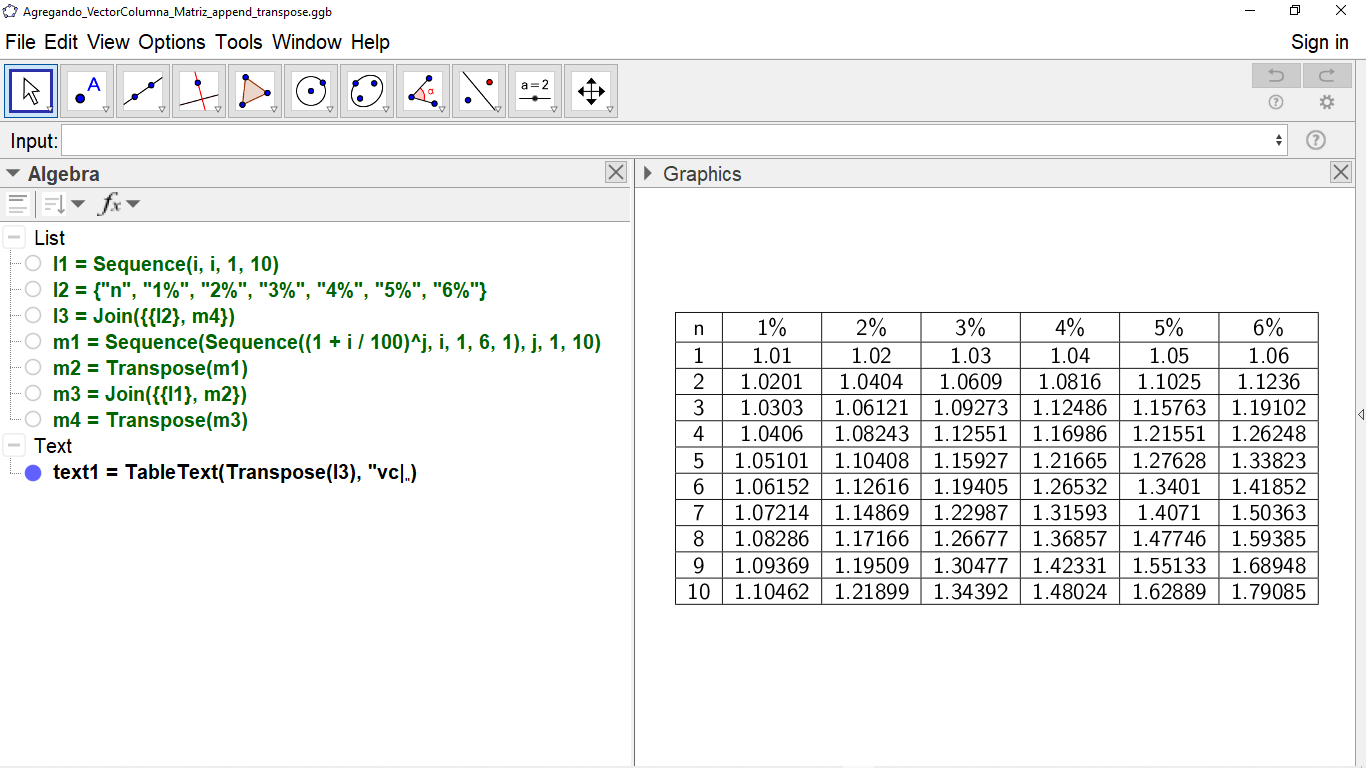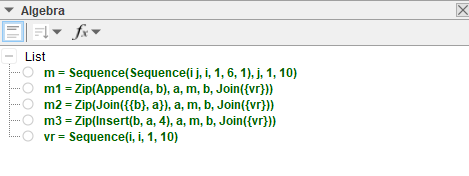# How to attach a column vector to a column and row matrix

jospercomp shared this question 2 years ago

How to attach a column vector to a matrix row column at the beginning or end of the column in the matrix

vr=Sequence(i, i, 1, 10)

vc=Zip({p}, p, l1)m1=Sequence(Sequence(i j, i, 1, 6, 1), j, 1, 10)@jospercomp Why is your row vector a simple list? For consistency (and better visualization) you should rather use a list containing a single list, i.e., something like {{1,2,3,4}}.

So here are some options in a bit more detail:

• Append(m, Join(vr)) -> adds after last row
• Join({m, vr}) -> adds after last row
• Join({vr, m}) -> adds before first row
• Insert(vr, m, j) -> inserts at row j

Adding the row vector as a matrix column can be done similarly to all the row versions above, just use Transpose(m) instead of m and also put the whole thing in another Transpose, e.g. Transpose(Append(Transpose(m), Join(vr))) and so on.

Alternatively, adding as column can be done without the double Transpose but with the use of Zip instead (works again for all the versions above):

• Zip(Append(a, b), a, m, b, Join(vr)) -> adds after last column
• Zip(Join({a, {b}}), a, m, b, Join(vr)) -> adds after last column
• Zip(Join({{b}, a}), a, m, b, Join(vr)) -> adds before first column
• Zip(Insert(b, a, j), a, m, b, Join(vr)) -> inserts at column j

When working with a column vector (matrix with one column) instead, everything can be done similarly. All the versions above that use Join(vr) can be used exactly the same with Join(vc) instead, because Join basically does the same for row and column vectors. For the other versions you can just replace vr either by Transpose(vc) or by {Join(vc)}.

And I am sure there are other possibilites as well, using a variety of list commands.2

ein bisschen verwirrend dein anliegen. (eine matrix besteht immer aus zeilen und spalten?)

um eine matrix um eine spalte zu erweitern musst du transponieren append, join eine zeile und wieder zurück transponieren.

zu matrix operationen siehe

https://www.geogebra.org/m/BpqJ28eP1

To add a column to a matrix, what commands should I use to transpose, add and join a "first or last" row and transpose again.

https://wiki.geogebra.org/e...1

Append( matrix, column vector in row) working fine but Append(, column vector in row.matrix) is not working correctly.2

@jospercomp Why is your row vector a simple list? For consistency (and better visualization) you should rather use a list containing a single list, i.e., something like {{1,2,3,4}}.

So here are some options in a bit more detail:

• Append(m, Join(vr)) -> adds after last row
• Join({m, vr}) -> adds after last row
• Join({vr, m}) -> adds before first row
• Insert(vr, m, j) -> inserts at row j

Adding the row vector as a matrix column can be done similarly to all the row versions above, just use Transpose(m) instead of m and also put the whole thing in another Transpose, e.g. Transpose(Append(Transpose(m), Join(vr))) and so on.

Alternatively, adding as column can be done without the double Transpose but with the use of Zip instead (works again for all the versions above):

• Zip(Append(a, b), a, m, b, Join(vr)) -> adds after last column
• Zip(Join({a, {b}}), a, m, b, Join(vr)) -> adds after last column
• Zip(Join({{b}, a}), a, m, b, Join(vr)) -> adds before first column
• Zip(Insert(b, a, j), a, m, b, Join(vr)) -> inserts at column j

When working with a column vector (matrix with one column) instead, everything can be done similarly. All the versions above that use Join(vr) can be used exactly the same with Join(vc) instead, because Join basically does the same for row and column vectors. For the other versions you can just replace vr either by Transpose(vc) or by {Join(vc)}.

And I am sure there are other possibilites as well, using a variety of list commands.1
• It is incorrect: Append(m, Join(vr)) -> adds after last row
• Is is correct: Append(m1, Join(vc)) -> adds after last row
• It is incorrect: Join({m, vr}) -> adds after last row
• It is correct: Join({m1, {vr}}) -> adds after last row
• It is incorrect: Join({vr, m}) -> adds before first row
• It is correct: Join({{vr}, m1}) -> adds before first row
• It is incorrect: Insert(vr, m, j) -> inserts at row j
• It is correct: Insert({vr}, m1, j) -> inserts at row j11

The reason you consider some versions incorrect is because your idea of a "row vector" is to use a simple list while I used a list of lists (a matrix with a single row) which I think is more consistent when using matrix-vector-terminology (see the first paragraph of my original answer), i.e. I used {{1,2,3,4}} instead of your {1,2,3,4}.

If you want to use a simple list for vr then of course you have to replace vr by {vr} in those versions (or just Join(vr) by vr in the cases where that is used).1

Thank you very much for all your advice and recommendations. I don't use the Join and Insert commands much. Sometimes I use Zip and usually use Append and Transpose1
• Zip(Append(a, b), a, m, b, Join({vr})) -> adds after last column
• Zip(Join({{b}, a}), a, m, b, Join({vr})) -> adds before first column
• Zip(Insert(b, a, j), a, m, b, Join({vr})) -> inserts at column j